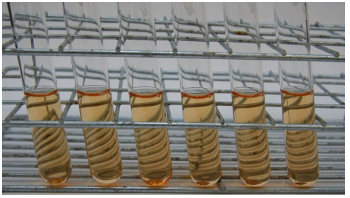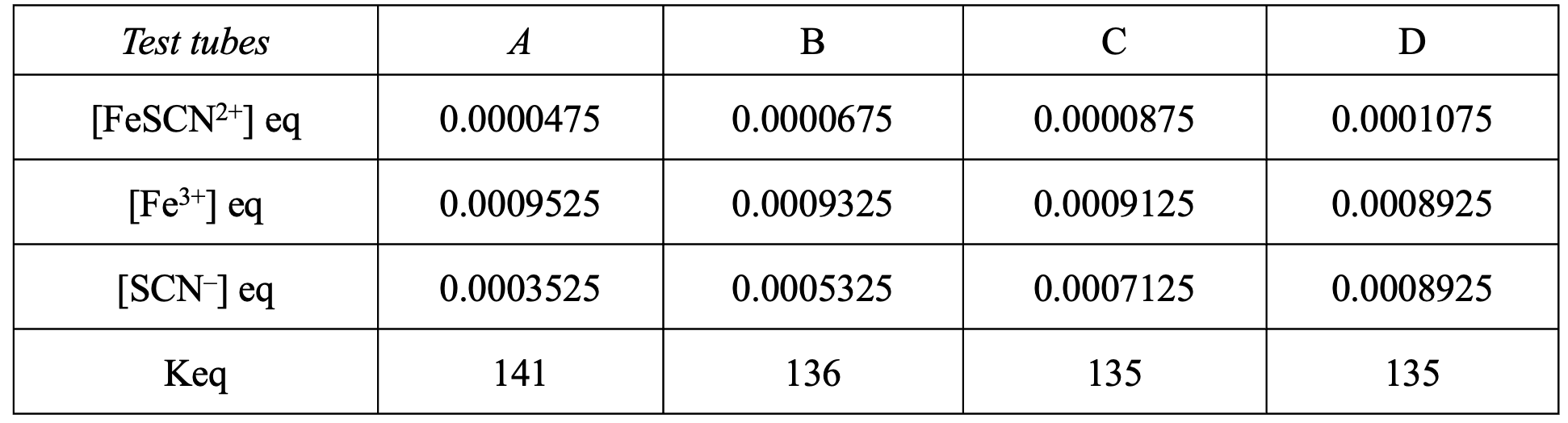# Using Colourimetry to Calculate Equilibrium Constant

This is part of the HSC Chemistry course under the topic Calculating Equilibrium Constant

### HSC Chemistry Syllabus

• conduct an investigation to determine Keq of a chemical equilibrium system, for example:

Keq of the iron(III) thiocyanate equilibrium (ACSCH096)

### What is Colourimetry?

Colourimetry is an analytical technique widely used in chemistry to measure the concentration of a coloured substance in a solution. One application of this method is to determine the equilibrium constant of a reaction. In this article, we will focus on the use of colorimetry to calculate the equilibrium constant for the formation of iron thiocyanate, a common topic in HSC Chemistry courses.

### The Iron(III) Thiocyanate System

The reaction of interest is the formation of iron thiocyanate complex, which is a reversible reaction:

$$Fe^{3+} (aq) + SCN^- (aq) ⇌ FeSCN^{2+} (aq)$$

The equilibrium constant, Keq, for this reaction can be determined by measuring the concentration of each species at equilibrium.

### Using Colorimetry to Determine Concentrations

Colorimetry relies on the principle that the absorbance of light by a coloured solution is proportional to the concentration of the coloured substance. In our case, the coloured substance is the iron thiocyanate complex (FeSCN^{2+}), which has a deep red colour.

The relationship between absorbance and concentration is described by the Beer-Lambert Law:

$$A = εcl$$

Where A is the absorbance, ε is the molar absorptivity, c is the concentration, and l is the path length (usually 1 cm).

To use colorimetry for determining the concentration of FeSCN^{2+}, follow these steps:

1. Prepare a series of standard solutions: Create a range of FeSCN^{2+} solutions with known concentrations by mixing known volumes of Fe^{3+} and SCN^- solutions. Make sure to use the same total volume for each solution to maintain consistency.2. Measure the absorbance: Use a colorimeter or spectrophotometer to measure the absorbance of each standard solution at a specific wavelength (typically around 447 nm for FeSCN^{2+}). Make sure to set the instrument to zero absorbance using a blank solution (i.e., only water without FeSCN^{2+}).3. Create a calibration curve: Plot the absorbance values against the known concentrations of FeSCN^{2+} in your standard solutions. This curve will allow you to determine the concentration of FeSCN^{2+} in an unknown solution based on its absorbance.

###Figure 1: Calibration curve showing the absorbance value of various standard solutions of iron(III) thiocyanate.

4. Measure the absorbance of the equilibrium mixture: Prepare an equilibrium mixture by mixing known volumes of Fe^{3+} and SCN^{-} solutions and allowing the reaction to reach equilibrium. Measure the absorbance of this mixture using the same wavelength as before.

5. Determine the concentration of FeSCN^{2+}: Use the calibration curve to find the concentration of FeSCN^{2+} in the equilibrium mixture based on its absorbance.

Table 1: equilibrium concentrations determined from measuring the absorbance of each iron(III) thiocyanate equilibrium mixture.### Calculating the Equilibrium Constant

Now that you have determined the concentration of FeSCN^{2+} at equilibrium, you can calculate the equilibrium constant, K_{eq}. To do this, you also need to determine the concentrations of Fe^{3+} and SCN^- at equilibrium.

Since you know the initial concentrations of Fe^{3+} and SCN^- and the amount of FeSCN^{2+} formed, you can calculate the concentrations of the reactants at equilibrium:

$$[Fe^{3+}]_{eq} = [Fe^{3+}]_{initial} - [FeSCN^{2+}]_{eq}$$

$$[SCN^-]_{eq} = [SCN^-]_{initial} - [FeSCN^{2+}]_{eq}$$

With these concentrations, you can now calculate the equilibrium constant:

$$K_{eq} = \frac{[FeSCN^{2+}]_{eq}}{[Fe^{3+}]_{eq} \times [SCN^-]_{eq}}$$

Table 2: equilibrium constant is calculated using equilibrium concentrations.Previous section: Calculating Reaction Quotient, Effect of Temperature on Equilibrium

Next section: Gibbs Free Energy and Equilibrium (Extension)